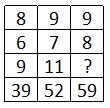Question 11

# Find out the missing number.Solution

The pattern followed here is,

8 x 6 - 9 = 39,

9 x 7 - 11 = 52,

9 x 8 - 13 = 59.

Hence, option D is the correct answer.

• Free SSC Study Material - 18000 Questions
• 230+ SSC previous papers with solutions PDF